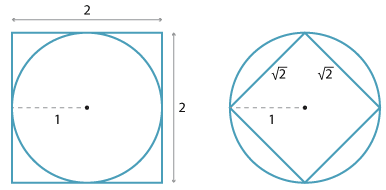### The real numbers and the rational numbers

We have seen that as we place halves, thirds, quarters, fifths, $$\ldots$$ on the number line, the maximum gap between successive fractions tends to zero. Thus if α is any real number then rational numbers can be placed increasingly close to α. Thus we can use rational numbers to approximate a real number correct to any required order of accuracy.Detailed description

For example, the diagrams above show a circle of area $$\pi$$ enclosed in a square of area 4, and enclosing a square of area 2, which proves that 2 < $$\pi$$ < 4.

Archimedes improved greatly on this result by using regular polygons with 96 sides, and was able to prove that $$3\dfrac{10}{71}<\pi<3\dfrac{1}{7}$$.